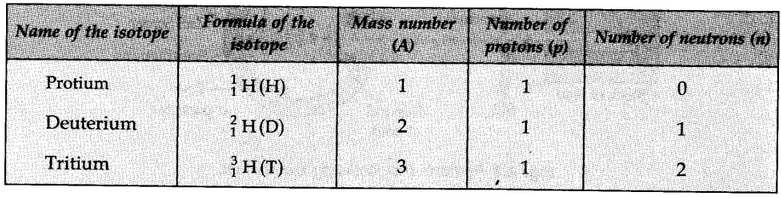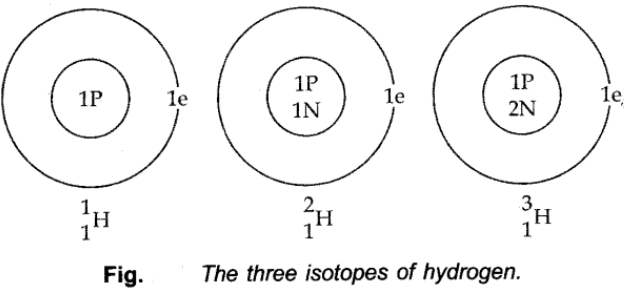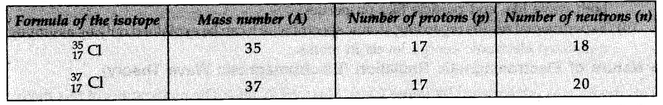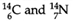# Atomic Number and Mass Number

## Atomic Number (Z):

The number of protons present in the nucleus is equal to the atomic number (Moseley 1913).

Atomic Number (Z)= Number of protons in the nucleus of an atom = Number of electrons in a neutral atom

Example:

• The number of protons in the hydrogen nucleus is 1, in sodium atom, it is 11, therefore, their atomic number are 1 and 11.
• In order to keep the electrical neutrality, the number of electrons in an atom is equal to the number of protons (atomic number, Z).
• For example, the number of electrons in a hydrogen atom and sodium atom are 1 and 11 respectively.

## Mass Number (A):

Sum of the number of protons and neutrons present in the nucleus.

Mass Number (A) = Number of protons (p) + Number of neutrons (n).

# Isotopes

Atoms with an identical atomic number but a different atomic mass number are known as Isotopes.

## Isotopes of Hydrogen:These three isotopes as shown in the figure below:## Isotopes of Chlorine:

These are two isotopes of chlorine with mass number 35 and 37.The two isotopes differ in their number of neutrons, having 18 and 20 neutrons, respectively.

# Isobars

• Isobars are the atoms with the same mass number but the different atomic number.

For example,• Each of these has the same mass number but a different atomic number.